## Rational Double Point

There are nine possible types of Isolated Singularities on a Cubic Surface, eight of them rational double points. Each type of Isolated Singularity has an associated normal form and Coxeter-Dynkin Diagram (,,,,,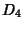,,and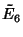).

The eight types of rational double points (thetype being the one excluded) can occur in only 20 combinations on a Cubic Surface (of which Fischer 1986 gives 19):,,,,,,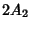,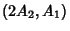,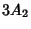,,,,, (),,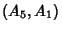,,, and(Looijenga 1978, Bruce and Wall 1979, Fischer 1986).

In particular, on a Cubic Surface, precisely those configurations of rational double points occur for which the disjoint union of the Coxeter-Dynkin Diagram is a Subgraph of the Coxeter-Dynkin Diagram. Also, a surface specializes to a more complicated one precisely when its graph is contained in the graph of the other one (Fischer 1986).

References

Bruce, J. and Wall, C. T. C. On the Classification of Cubic Surfaces.'' J. London Math. Soc. 19, 245-256, 1979.

Fischer, G. (Ed.). Mathematical Models from the Collections of Universities and Museums. Braunschweig, Germany: Vieweg, p. 13, 1986.

Fischer, G. (Ed.). Plates 14-31 in Mathematische Modelle/Mathematical Models, Bildband/Photograph Volume. Braunschweig, Germany: Vieweg, pp. 17-31, 1986.

Looijenga, E. On the Semi-Universal Deformation of a Simple Elliptic Hypersurface Singularity. Part II: The Discriminant.'' Topology 17, 23-40, 1978.

Rodenberg, C. Modelle von Flächen dritter Ordnung.'' In Mathematische Abhandlungen aus dem Verlage Mathematischer Modelle von Martin Schilling. Halle a. S., 1904.